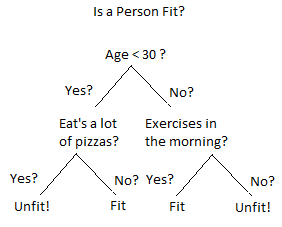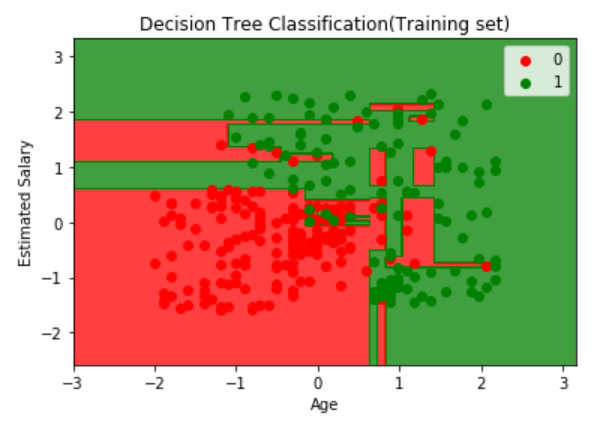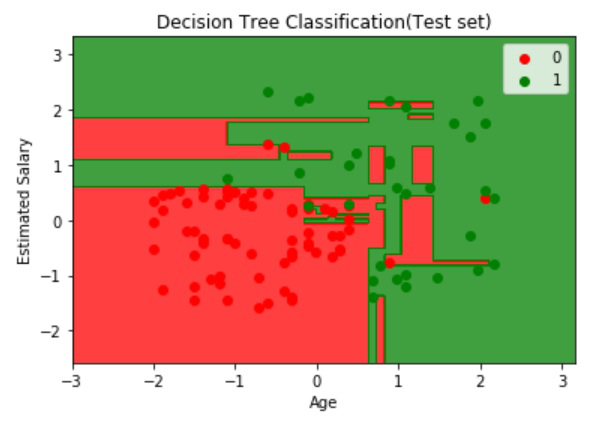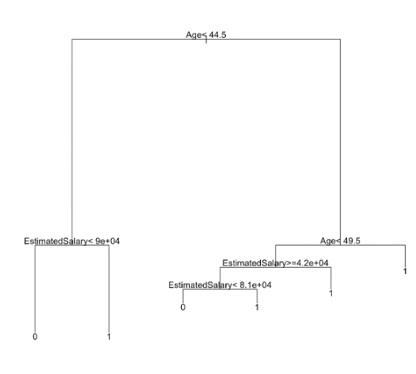# Decision Tree Classifiers in R Programming

Classification is the task in which objects of several categories are categorized into their respective classes using the properties of classes. A classification model is typically used to,

• Predict the class label for a new unlabeled data object
• Provide a descriptive model explaining what features characterize objects in each class

There are various types of classification techniques such as,

## Decision Tree Classifiers in R Programming

A decision tree is a flowchart-like tree structure in which the internal node represents feature(or attribute), the branch represents a decision rule, and each leaf node represents the outcome. A Decision Tree consists of,

• Nodes: Test for the value of a certain attribute.
• Edges/Branch: Represents a decision rule and connect to the next node.
• Leaf nodes: Terminal nodes that represent class labels or class distribution.And this algorithm can easily be implemented in the R language. Some important points about decision tree classifiers are,

• It is more interpretable
• Automatically handles decision-making
• Bisects the space into smaller spaces
• Prone to overfitting
• Can be trained on a small training set
• Majorly affected by noise

## Implementation in R

### The Dataset:

A sample population of 400 people shared their age, gender, and salary with a product company, and if they bought the product or not(0 means no, 1 means yes). Download the dataset Advertisement.csv.

## R

 `# Importing the dataset` `dataset = ``read.csv``(``'Advertisement.csv'``)` `head``(dataset, 10)`

Output:

### Train the data

To train the data we will split the dataset into a test set and then make Decision Tree Classifiers with rpart package.

## R

 `# Encoding the target feature as factor` `dataset\$Purchased = ``factor``(dataset\$Purchased, ` `                           ``levels = ``c``(0, 1))`   `# Splitting the dataset into ` `# the Training set and Test set` `# install.packages('caTools')` `library``(caTools)` `set.seed``(123)` `split = ``sample.split``(dataset\$Purchased, ` `                     ``SplitRatio = 0.75)` `training_set = ``subset``(dataset, split == ``TRUE``)` `test_set = ``subset``(dataset, split == ``FALSE``)`   `# Feature Scaling` `training_set[-3] = ``scale``(training_set[-3])` `test_set[-3] = ``scale``(test_set[-3])`   `# Fitting Decision Tree Classification ` `# to the Training set` `# install.packages('rpart')` `library``(rpart)` `classifier = ``rpart``(formula = Purchased ~ .,` `                   ``data = training_set)`   `# Predicting the Test set results` `y_pred = ``predict``(classifier, ` `                 ``newdata = test_set[-3], ` `                 ``type = ``'class'``)`   `# Making the Confusion Matrix` `cm = ``table``(test_set[, 3], y_pred)`

• The training set contains 300 entries.
• The test set contains 100 entries.
```Confusion Matrix:
[[62,  6],
[ 3, 29]]```

## R

 `# Visualising the Training set results` `# Install ElemStatLearn if not present ` `# in the packages using(without hashtag)` `# install.packages('ElemStatLearn')` `library``(ElemStatLearn)` `set = training_set`   `# Building a grid of Age Column(X1) ` `# and Estimated Salary(X2) Column` `X1 = ``seq``(``min``(set[, 1]) - 1, ` `         ``max``(set[, 1]) + 1, ` `         ``by = 0.01)` `X2 = ``seq``(``min``(set[, 2]) - 1,` `         ``max``(set[, 2]) + 1,` `         ``by = 0.01)` `grid_set = ``expand.grid``(X1, X2)`   `# Give name to the columns of matrix` `colnames``(grid_set) = ``c``(``'Age'``,` `                       ``'EstimatedSalary'``)`   `# Predicting the values and plotting them ` `# to grid and labelling the axes` `y_grid = ``predict``(classifier, ` `                 ``newdata = grid_set,` `                 ``type = ``'class'``)` `plot``(set[, -3],` `     ``main = 'Decision Tree ` `             ``Classification ``(Training set)',` `     ``xlab = ``'Age'``, ylab = ``'Estimated Salary'``,` `     ``xlim = ``range``(X1), ylim = ``range``(X2))` `contour``(X1, X2, ``matrix``(``as.numeric``(y_grid),` `                       ``length``(X1),` `                       ``length``(X2)), ` `                       ``add = ``TRUE``)` `points``(grid_set, pch = ``'.'``, ` `       ``col = ``ifelse``(y_grid == 1, ` `                    ``'springgreen3'``,` `                    ``'tomato'``))` `points``(set, pch = 21, bg = ``ifelse``(set[, 3] == 1, ` `                                  ``'green4'``, ` `                                  ``'red3'``))`

Output:## R

 `# Visualising the Test set results` `library``(ElemStatLearn)` `set = test_set`   `# Building a grid of Age Column(X1) ` `# and Estimated Salary(X2) Column` `X1 = ``seq``(``min``(set[, 1]) - 1, ` `         ``max``(set[, 1]) + 1,` `         ``by = 0.01)` `X2 = ``seq``(``min``(set[, 2]) - 1,` `         ``max``(set[, 2]) + 1,` `         ``by = 0.01)` `grid_set = ``expand.grid``(X1, X2)`   `# Give name to the columns of matrix` `colnames``(grid_set) = ``c``(``'Age'``, ` `                       ``'EstimatedSalary'``)`   `# Predicting the values and plotting them` `# to grid and labelling the axes` `y_grid = ``predict``(classifier,` `                 ``newdata = grid_set,` `                 ``type = ``'class'``)` `plot``(set[, -3], main = 'Decision Tree ` `                        ``Classification ``(Test set)',` `     ``xlab = ``'Age'``, ylab = ``'Estimated Salary'``,` `     ``xlim = ``range``(X1), ylim = ``range``(X2))` `contour``(X1, X2, ``matrix``(``as.numeric``(y_grid),` `                       ``length``(X1), ` `                       ``length``(X2)),` `                       ``add = ``TRUE``)` `points``(grid_set, pch = ``'.'``, ` `       ``col = ``ifelse``(y_grid == 1, ` `                    ``'springgreen3'``,` `                    ``'tomato'``))` `points``(set, pch = 21, bg = ``ifelse``(set[, 3] == 1,` `                                  ``'green4'``,` `                                  ``'red3'``))`

Output:## R

 `# Plotting the tree` `plot``(classifier)` `text``(classifier)`

Output:Whether you're preparing for your first job interview or aiming to upskill in this ever-evolving tech landscape, GeeksforGeeks Courses are your key to success. We provide top-quality content at affordable prices, all geared towards accelerating your growth in a time-bound manner. Join the millions we've already empowered, and we're here to do the same for you. Don't miss out - check it out now!

Previous
Next